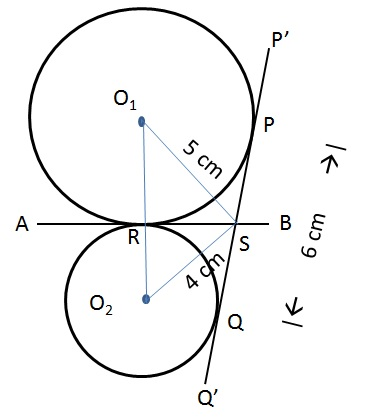# CAT Quantitative Aptitude Questions | Geometry Questions for CAT - Triangles

###### CAT Questions | CAT Geometry Questions | Circles and Triangle

The question is from CAT Geometry - Triangles. It tests our understanding on properties of a triangle. CAT Geometry questions are heavily tested in CAT exam. Make sure you master Geometry problems. In this question it discusses about the properties of area of a triangle.

Question 30: Two circles with centres O1 and O2 touch each other externally at a point R. AB is a tangent to both the circles passing through R. P’Q’ is another tangent to the circles touching them at P and Q respectively and also cutting AB at S. PQ measures 6 cm and the point S is at distance of 5 cms and 4 cms from the centres of the circles. What is the area of the triangle SO1O2?

1. 9 cm2
2. 3(4+√7)/2 cm2
3. 27/2 cm2
4. (3√41)/2 cm2

## Best CAT Coaching in Chennai

#### CAT Coaching in Chennai - CAT 2022Limited Seats Available - Register Now!

##### Method of solving this CAT Question from Triangles: To calculate the area of a triangle, determine its base and altitude!!From the diagram we see that SP, SR are tangents to circle1 from same point S. Similarly SR, SQ are tangents from same point to circle 2.
SP = SR; SQ = SR implies SP = SQ
Given PQ = 6cm
SP + SQ = 6
Therefor SR = SP = SQ = 3 cm.

SR is the altitude to the triangle SO1O2. We need to find the length of the base O1O2 to determine the area.
O1RS is a right angled triangle with hypotenuse = 5 and one side = 3
Therefore, O1R = √(52 - 32) = 4cm
Similarly, O2RS is a right angled triangle with hypotenuse = 4 and one side = 3
Therefore, O2R = √(42 - 32) = √7cm
O1O2 = O1R + O2R = 4 + √7
Area of the triangle SO1O2 = 1/2 * SR * O1O2 = 1/2 * 3 * (4 + √7) cm2

The question is " What is the area of the triangle SO1O2?"

##### Hence, the answer is 3(4+√7)/2 cm2.

Choice B is the correct answer.

###### CAT Coaching in ChennaiCAT 2023

Classroom Batches Starting Now! @Gopalapuram

###### Best CAT Coaching in Chennai Introductory offer of 5000/-

Attend a Demo Class

## CAT Preparation Online | CAT Geometry questions Videos On YouTube

#### Other useful sources for Geometry Question | Geometry Triangles Circles Quadrilaterals Sample Questions

##### Where is 2IIM located?

2IIM Online CAT Coaching
A Fermat Education Initiative,
58/16, Indira Gandhi Street,
Kaveri Rangan Nagar, Saligramam, Chennai 600 093

##### How to reach 2IIM?

Phone: (91) 44 4505 8484
Mobile: (91) 99626 48484
WhatsApp: WhatsApp Now
Email: prep@2iim.com